PHYSICS

Mass

UNIVERSE

UA. Kong Gravitational Force UB. Sun UC. Earth
UE. Black Hole UF. Life of Universe UG. Properties of Universe UH. Kong Matter and Kong Energy

UA. KONG GRAVITATIONAL FORCE

1. Gravitational Force 2. Magnetic Force 3. Electric Force 4. Kong Gravitational Force
5. Attraction Force Between Sun and Earth 6. Effect of Kong Gravitational Force 7. Kong Gravitational Force in Galaxies 8. Relationship Between Kong and Newtonian Gravitational Force

INTRODUCTION

In this chapter, an alternative understanding on the gravitational force is introduced. We will discuss about the actual forces that govern the gravitational force. The gravitational force is redefined based on the actual force instead of mass. Explanations and descriptions are given to support this alternative understanding.

If the Newtonian gravitational force governs the Universe, the Universe shall be spherical in shape since the Big Bang. However, it is not. The Universe is expanding in elongated shape which is caused by the Kong gravitational forces.

Although many theories had been developed using the gravitational force, these theories can be modified base on the actual attraction force.

OBJECTIVES

1)      To discuss about the mass dependent gravitational force.

2)      To describe the alternative understanding about the attraction forces.

3)      To explain the forces those contribute to the attraction forces.

4)      To develop the magnetic and electric forces.

5)      To give supportive circumstances on the attraction forces.

6)      To discuss on the attraction forces between the Sun and the Earth.

7)      To discuss on the forces that cause the Universe to be planar instead of spherical.

## UA.1.0        GRAVITATIONAL FORCE

Does the mass dependent gravitational force govern the activities of the whole universe? Does the gravitational force cause the apple to fall on the earth? Does the gravitational force pull everything onto the earth? Does the ‘massive’ sun gravitationally pull all the planets circulating around it? Why the planets are circulating around the stars but not planets circulate to planets?

Although the Newton’s law of universal gravitation had been adopted for many years and Einstein had also once modified the theory using general relativity principle, the author still would like to share an alternative understanding. There are still some unexplained circumstances based on the mass dependent gravitational force model.

The charges have positive and negative potential, the magnet has north and south potential, which able to attract and repulse each other. When there is positive, the negative must exist. When there is attraction, the repulsive must exist. Gravitational force is a uni-direction force which has only attractive but without repulsive force. There is no anti-matter or anti-gravitational force. Furthermore, light is mass-less and it does not make sense that light can be attracted base on the mass dependent gravitational law. This is one of the discrepancies of the Newton's law of gravitational force that triggers the mindset of the author.

If the gravitational force pulls the Earth, why not the North Pole of the Earth faces the Sun? Why is the axis of rotation of the earth tilting? What cause the tilting effect? Why and how the earth possesses the strong magnetic field that protecting the earth from the solar wind?

If the Newton's law of gravitational force pulls all the objects in the Universe, shall the Universe grow in spherical shape? as this gravitational force has no preference in pulling objects from any direction. However, it is not, this is one of the biggest discrepancies for Newton's law of gravitational force. Many galaxies are flat in shape which is also a discrepancy for Newton's law of gravitational force.

Another discrepancy is some objects are observed that moving away from us in acceleration. If the Newton's law of gravitational force exacts a pulling force to all objects, the objects shall moving away in deceleration.

Base on these discrepancies, the arbitrary theory on Newton's law of gravitational force is challenged. Shall we open up our view to an alternative theory on Kong Gravitational Force.

UA.1.1        Attraction Forces

According to the understanding of the author, there are only two forces exist in the universe since the beginning of universe. There are the magnetic and electric (M&E) forces only.

Referring to the topics on the ‘Fundamental of Physics’ and the ‘Mass’, it is dissected and derived that mass is a function of magnetic, charge and time. Mass is made up of nothing but the M&E field as presented and described in the chapters about mass.

Since mass can be fundamentalized into the three parameters, the mass dependent gravitational force can also be dissected to the M&E forces. The general theory and the understanding is, it is the magnetic and electric forces that govern the attraction force, but not the mass dependent gravitational force.

UA.2.0        MAGNETIC FORCE

Each atom is a magnetic dipole. At very low temperature where the atom is not vibrating violently but freely moving, each atom dipole is able to attract each other through magnetic force to form a bigger magnet bar at lower total combined energy. The formation of magnet bar produces a stronger external superimposed magnetic field. This is the uni-direction of attraction force that forms parts of the gravitational force.

For the moving charged particle in magnetic field, the magnetic force acts on the charged particle can be written as follow,

FB = qv ´ B                                                                                         … eq. UA.2.1

where   q = charge of charged particle

v = velocity of charged particle

B = magnetic field

The moving charged particle is able to produce magnetic field. This induced magnetic field can be expressed by the Ampere’s Law shown below,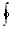B×ds = moi                                                                                       … eq. UA.2.2

where   mo = permeability constant

i = current

ds = distance in vector

Ampere’s law is applied to a closed loop called an Amperian loop. The circle on the integral sign indicates that the quantity B×ds is integrated around the closed loop.B×ds = mo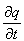… eq. UA.2.3

From the Amperian loop, we multiple the vector dx on both sides to produce a finite surface as shown in figure UA.2.1 below.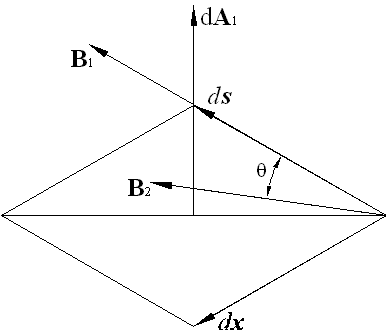Figure UA.2.1

Eq. UA.2.3 then becomes,(B×ds)dx = modx                                                                       … eq. UA.2.4

From the vector transformation, we have,

B ´ (ds ´ dx) = (B×dx)ds – (B×ds)dx                                                  … eq. UA.2.5

Rearrange eq. UA.2.5, we obtain,

(B×ds)dx = (B×dx)dsB ´ (ds ´ dx)                                                  … eq. UA.2.6

Substitute eq. UA.2.6 into eq. UA.2.4, we obtain,(B×dx)dsB ´ (ds ´ dx) = modx                                             ... eq. UA.2.7

Rearrange and simplify eq. UA.2.7, we obtain,B ´ dA = moqv                                                                              … eq. UA.2.8

where   B×dx = 0 (dx is always selected that it is perpendicular to B)

dA = ds ´ dx

v  = dx/dt

idx = qv

The integration of eq. UA.2.8 is integrated around the hoop with the width dx. Substitute eq. UA.2.8 into eq. UA.2.1, we obtain,

dFB =(B1 ´ dA1) ´ B2                                                            … eq. UA.2.9

dFB =B2 ´ (B1 ´ dA1)                                                             ... eq. UA.2.10

Using the vector transformation, eq. UA.2.10 becomes,

dFB =(B2× dA1)B1 – (B2 × B1)dA1                                              ... eq. UA.2.11

Integral eq. UA.2.11 to a Gaussian surface, we obtain,

FB = ò dFB =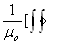(B2× dA1)B1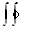(B2 × B1)dA1 ]                      ... eq. UA.2.13

Rearrange and simplify eq. UA.2.12, we obtain,

FB = –(B2 × B1)dA1                                                                  ... eq. UA.2.14

whereB×dA = 0 (Gauss’s law for magnetism, the net magnetic flux through any closed Gaussian surface must be zero.)

Therefore, the magnetic force is expressed as follow,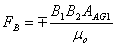… eq. UA.2.15

where   B1 = magnetic field 1

B2 = magnetic field 2

AAG1 = Amperian-Gaussian surface 1

mo = permeability constant

The direction of magnetic field is depending on clockwise or anti-clockwise. Therefore, the negative sign shows the both are anti-clockwise direction and vice versa. The negative sign on the eq. UA.2.15 shows the attraction force.

UA.2.1        Wire Carrying Current

Figure UA.2.2 below shows the two wires carrying current at the same direction. Two wires are separated apart with distance ‘d’ and the length of the wires is ‘L’. Wire 1 carries current i1 and wire 2 carries current i2. The magnetic field generated by wire 1 is B1 and B2 is generated by wire 2. The enclosed Gaussian surface is the cylindrical surface with radius ‘d’ and length ‘L’. The B1 intersect with B2 at two locations with the angle 60°.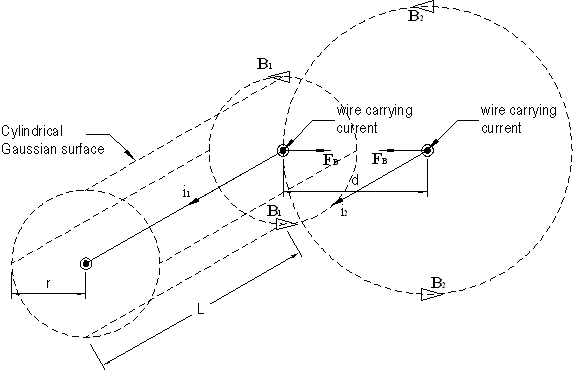Figure UA.2.2

We now to find the magnetic force generated between the two wires carrying current. The magnetic field generated by the wire 1 with the current i1 is given by the ampere’s law as follow,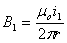(anti-clockwise)                                                                  … eq. UA.2.16

and magnetic field generated by wire 2 is(anti-clockwise)                                                                 … eq. UA.2.17

The cylindrical Gaussian surface is

AAG1 = 2prL                                                                                        … eq. UA.2.18

The end surface of the cylinder is perpendicular to the magnetic field and it is negligible for the long straight wires.

Substitute eq. UA.2.16, eq. UA.2.17 and eq. UA.2.18 into eq.UA.2.15, we obtain,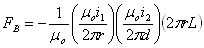… eq. UA.2.19

The magnetic force between the two wires is given by eq. UA.2.19. The negative sign shows the attraction force. The two wires are attracted to each other.

UA.2.2        Magnetic Force to Magnetic Dipole

For magnetic force exerts to magnetic dipole, the force is developed using a different model. As described in the chapter “Kong Atom Model”, all the substances or objects in this universe are magnetic dipole. All the atoms and electrons are magnetic dipole. The magnetic dipole characteristic can be represented with the current loop. A current loop is shown in figure UA.2.3. The current loop produces magnetic field ‘B’ with current ‘i’. The shape of the magnetic field produced is the inner merged toroid. The distance from the current loop to the magnetic field is r.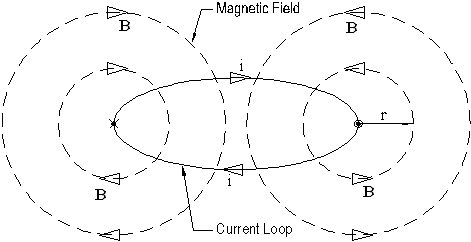Figure UA.2.3

The magnetic energy to the magnetic dipole is

U = - m × Bext                                                                                       … eq. UA.2.20

where   m = magnetic dipole

Bext = external magnetic field

From the Ampere’s law, we can express the magnetic field of the current loop at the outer side as follow,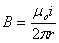… eq. UA.2.21

where   mo = permeability constant

i = current carrying by the imaginative wire

r = distance from the centre of the imaginative wire

Assume that all the magnetic dipoles tend to line in the direction of the external magnetic field such as compass. The angle between the magnetic dipole and external magnetic field is always zero. The dot product of eq. UA.2.20 is always a unity. Substitute eq. UA.2.21 into UA.2.20, we obtain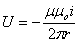… eq. UA.2.22

The alignment of the magnetic dipole to the external magnetic field always creates the attractive force. Therefore, we can express the magnetic energy in terms of magnetic force as follow,

U = - FB×dr                                                                                          … eq. UA.2.23

The attractive force of the current loop is always toward the current loop which align to distance r. Therefore, the dot product of eq. UA.2.23 is always a unity.

Equating eq. UA.2.22 and eq. UA.2.23, we obtain… eq. UA.2.24

Differentiate both sides of eq. UA.2.24 with dr, we obtain… eq UA.2.25

where   GB = mo/2p

Eq. UA.2.25 shows the magnetic force acts on a magnetic dipole. The negative sign shows the attractive force. The magnetic force is proportion to the reverse square of the distance.

Both eq. UA.2.15 and eq. UA.2.25 are the magnetic force. Both objects must possess certain magnetic property in order to be attracted by magnetic force. Since all the objects in this universe are magnetic dipole or current loop and able to rotate freely, they tend to attract to each other in order to achieve lower energy level. This is the cause of the uni-direction attraction force.

UA.2.3        Magnetic Force on Earth

The Earth possesses magnetic field. From the chapter ‘Earth’, it describes that the magnetic field is caused by the rotating effect and the net current in the Earth. Figure UA.2.4 shows the current loop of the Earth. The current loop produces the external magnetic field. When the magnetic dipole of the object is aligned with the external magnetic field, it will be attracted by the magnetic force of the Earth.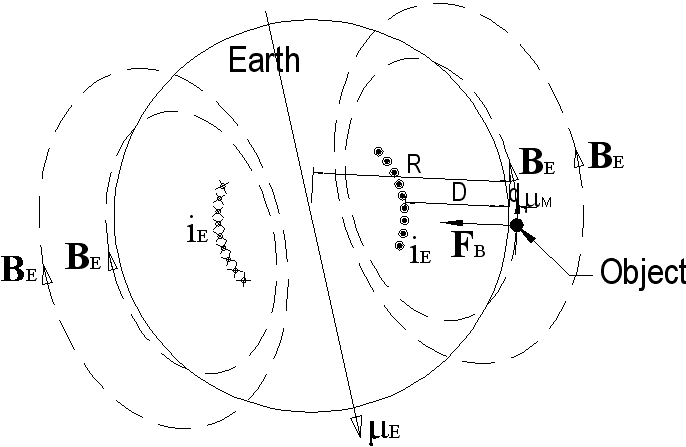Figure UA.2.4

The distance ‘r’ of eq. UA.2.25 is the distance from the object to the current loop. From figure UA.2.4, the relation of the distances is

r = D + d

r = kR + d                                                                                           … eq. UA.2.26

where   D = kR

Substituting eq. UA.2.26 into eq. UA.2.25, we obtain… eq. UA.2.27

where   mM = magnetic moment of object with mass M

iE = intrinsic current of the Earth that produce the magnetic field of BE

Expand eq. UA.2.27 by binomial theorem,… eq. UA.2.28

For the distance d < R, eq. UA.2.28  becomes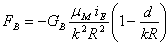… eq. UA.2.29

For an object near to the Earth surface, where d << R, the magnetic force is almost constant.… eq. UA.2.30

On the surface of the Earth, all the objects are tending to be attracted by the Earth in order to achieve lower energy level. The magnetic attraction force is proportional to the reverse square of the distance.

The magnetic field on the Earth surface is roughly 10-4 T, which is very weak. For a magnet bar having the mass of 50g with the magnetic dipole moment of 5 J/T, the magnetic attraction force on the Earth is very small and negligible.

UA.3.0        ELECTRIC FORCE

All the objects or mass have moving electrons. They can be either permanent or temporary charged electrostatically. The phenomenon of the electrostatic charged objects to the neutral objects is always attraction. This is the uni-direction of attractive force that contributes to another part of the gravitational force.

UA.3.1        Coulomb’s Law

The electrostatic force of attraction or repulsion between two charged particles that separated by a distance r is expressed by the Coulomb’s law as follow… eq. UA.3.1

where   q1 = charged particle 1

q2 = charged particle 2

r = distance between two particles

eo = permittivity constant

GE = 1/4peo = constant

When a charged object is brought near to a neutral object such as an isolated copper, the charged object will always attract the neutral object. The electrons of the neutral object will be attracted and concentrated at the point nearest distance to the charged object.

If the isolated copper is mild positive charged and a stronger positive charged object is brought near to the copper, the charged object will still attract the copper.

If two charged objects with different strength of charges brought near to the copper, the copper will be attracted nearer to the object with higher strength of charge.

UA.3.2        Dielectric and Conductor

All the mass appear in the universe can be a dielectric or conductor. Dielectric is a particle or object that able to produce electric dipole, either temporary or permanently. Since dielectric is able to produce electric dipole, it is able to react upon to the M&E field.

When a conductor is placed in an electric field, the electrons will be induced according to the direction of the electric field. The negative charges will be attracted nearer to the positive potential and vice versa. This phenomenon is also to reduce the external electric strength by storing the energy within itself.

The earth consists of both the dielectrics and conductors. Therefore, the Earth is able to be charged either temporary or permanently.

UA.3.3        Electric Force to Electric Dipole

Figure UA.3.1 shows an electric dipole due to the internal charge separation upon external electric force q1. The electrons are concentrated at one end with charge q2. The positive charges are distributed along the object and represented by an average charge q2 at distance d from the concentrated negative charge. This is because some of the electrons are able to move freely in the object. The distance between the external charge q1 to the concentrated negative charge q2 of the object is r.Figure UA.3.1

The resultant electric forces from the external charge to the object is expressed as follow,

FE = F1 – F2                                                                                      … eq. UA.3.2

Substitute eq. UA.3.1 into eq. UA.3.2, we obtain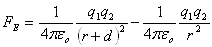… eq. UA.3.3

Rearrange eq. UA.3.3, we obtain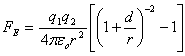… eq. UA.3.4

Then, we can expand eq. UA3.4 with the binomial theorem as follow,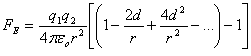… eq. UA.3.5

For distance d << r, we can ignore the higher term of the binomial expansion. Eq. UA.3.5 becomes,… eq. UA.3.6

where   p = q2d = electric dipole

In figure UA.3.1, the force balance between the external force and the internal force causes the electrons to stay inside the object. We can express the force equilibrium as follow,

F2 = F3                                                                                                … eq. UA.3.7

Substitute eq. UA.3.1 into eq. UA.3.7, we obtain,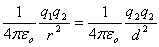… eq. UA.3.8

Rearrange eq. UA.3.8, we obtain,… eq. UA.3.9

Substitute eq. UA.3.9 into eq. UA.3.6, we obtain,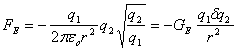… eq. UA.3.10

where   GE = 1 / 2peo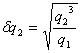Eq. UA.3.10 shows the electric force acts on an electric dipole. The negative sign shows the attractive force. The electric force is proportion to the reverse square of the distance.

If an object is able to form the electric dipole upon the external electric force, it will be attracted toward the external force to achieve lower energy level. This is the cause of the uni-direction attraction force.

UA.3.4        Electric Force between Two Electric Dipoles

Figure UA.3.2 shows the electric force between two electric dipoles. The first electric dipole consists of internal charge separation of q1n and q1p. The second electric dipole consists of concentrated electrons at one end with charge q2n and the distributed positive charge q2p. The distance between all the charges are a, b and c respectively.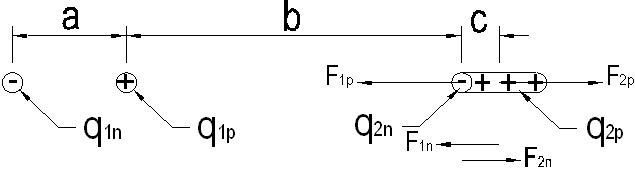Figure UA.3.2

The electric force acting on the dipole 1 to dipole 2 is as follow,

FE = – F1p + F2p – F1n + F2n                                                           ... eq. UA.3.11

Substitute eq. UA.3.1 into eq. UA.3.11, we obtain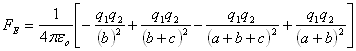… eq. UA.3.12

where   q1p = q1n = q1

q2p = q2n = q2

Rearrange eq. UA.3.12, we obtain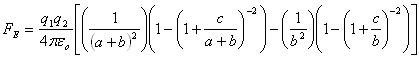… eq. UA.3.13

Then, we expand eq. UA3.13 with the binomial theorem as follow,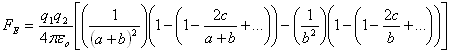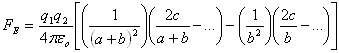… eq. UA.3.14

For distance c << a + b and c << b, we can ignore the higher term of the binomial expansion. Eq. UA.3.14 becomes,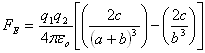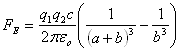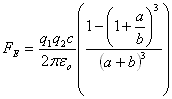… eq. UA.3.15

For the force balance on the concentrated electrons in the electric dipole 2, the force balance is shown in figure UA.3.3 below,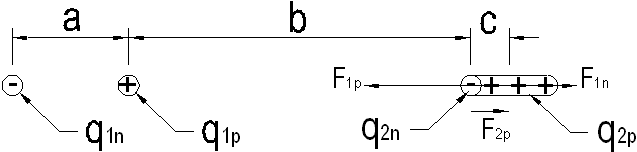Figure UA.3.3

We can express the force equilibrium as follow,

F1n + F2p – F1p = 0                                                                           … eq. UA.3.16

Substitute eq. UA.3.1 into eq. UA.3.13, we obtain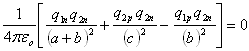… eq. UA.3.17

Rearrange eq. UA.3.17, we obtain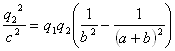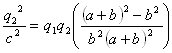… eq. UA.3.18

where   q1p = q1n = q1

q2p = q2n = q2

Rearrange to obtain the distance c,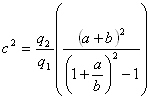… eq. UA.3.19

Substitute eq. UA.3.19 into eq. UA.3.15, we obtain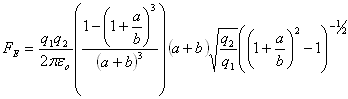… eq. UA.3.20

Rearrange eq. UA.3.20, we obtain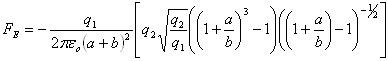… eq. UA.3.21

Simplify eq. UA.3.21, we obtain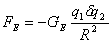… eq. UA.3.22

where   GE = 1 / 2peo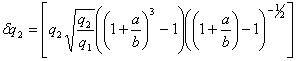a / b > 0

R = a + b

Eq. UA.3.22 shows the electric force acts by an electric dipole to another electric dipole. The negative sign shows the attractive force. The electric force is proportion to the reverse square of the distance.

UA.3.5        Electric Force on Earth

The Earth possesses electric field. From the chapter ‘The Earth’, it describes that the electric field is caused by the charges separation of the Earth. The outer layer of the Earth is induced to become positive charge and the inner of the Earth becomes negative charge. Figure UA.3.4 shows the electric field of the Earth and the object attracted to the Earth. When the electric dipole is aligned to the electric field of the Earth, it will be attracted to the Earth.Figure UA.3.4

The Earth surface possesses charge qE and the Earth electric field is outward from the Earth. Under the Earth electric field, the object becomes an electric dipole and produces the delta charge, qM. The distance from the object to the center of the Earth is R.

From eq. UA.3.22, the electric force of the Earth exerts on the object can be expressed as follow,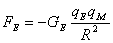… eq. UA.3.23

where   GE = 1 / 2peo

On the surface of the Earth, all the objects tend to be attracted by the Earth in order to achieve lower energy level. The electric attraction force is proportional to the reverse square of the distance.

UA.3.6        Approximation on the Earth Attraction Force

From the magnetic field on the Earth, we can approximately estimate the separated charges of the Earth. The estimation is shown below,… eq. UA.3.24

Substitute all the known constants into eq. UA.3.24, we obtain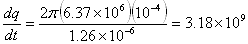… eq. UA.3.25

The separated charges of the Earth is

qE = ( 3.18´109 ) ´ 3600 ´ 24 = 2.74 ´ 1014                                      … eq. UA.3.26

Substitute eq. UA.3.26 into eq. UA.3.23, the approximate estimated electric attraction force of the Earth is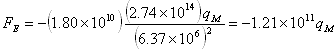… eq UA.3.27

On the Earth surface, the attraction force to an object having the mass of 1 kg is approximately 9.81 N. For the 1-kg mass, the induced delta charge of the object is

qM ≈ 8.1 ´ 10-11 C                                                                              … eq. UA.3.28

The induced delta charge of the object is reasonably accepted. Eq. UA.3.24 only shows the net current flow between the positive and negative charges of the Earth. In actual case, the separate charge of the Earth is much higher and the induced delta charge, qM of the object is much smaller and unnoticeable. It is difficult to measure the induced delta charge for the 1-kg mass because of the followings:-

1)      the Earth exerts the same electric field to all objects on the Earth.

2)      the distance between the induced charges of the electric dipole is short.

3)      the induced electric dipole is temporarily and appear in very short period due to vibrations.

UA.4.0        KONG GRAVITATIONAL FORCE

The mass dependent gravitational force is the Newton's law of gravitational force or the Newtonian gravitational force. The gravitational force caused by the magnetic and electric force is the Kong gravitational force. From the mass dependent gravitational force, it is expressed as follow,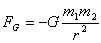… eq. UA.4.1

From the dimensional analysis in the chapter of “Fundamental of Physics”, we found that mass is a function of charge, magnetic and time, where

[M] = [Q][B][T]                                                                                   … eq. UA.4.2

where   [M] = dimension of mass

[Q] = dimension of charge

[B] = dimension of magnetic field

[T] = dimension of time

For simplest analysis, the function is separated to individual variables as follow,

M(q,B,t) = Q(q)B(b)T(t)                                                                      … eq. UA.4.3

The partial derivation equation to the respective dimensions can be expressed as follow,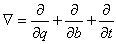… eq. UA.4.4

Inserting eq. UA.4.3 into eq. UA.4.4, we obtain,… eq. UA.4.5

Solving the eq. UA.4.5, we obtain,

ÑM = GG = GE + GB + GT                                                               … eq. UA.4.6

where   GG = Constant for mass component

GE = Constant for electric component

GB = Constant for magnetic component

GT = Constant for time component

Each variable is independent. If the mass property of an object is constant, the electric property, magnetic property and time property of the object is constant. The mass dependent gravitational force is related to the two M&E forces as follow,

FG = FE + FB                                                                                      … eq. UA.4.7

Substitute eq. UA.2.25, eq. UA.3.1 and UA.4.1 into eq. UA.4.7, we obtain,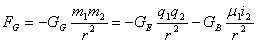… eq. UA.4.8

The negative sign in eq. UA.4.8 shows the attraction forces and always attraction as mentioned above.

Coincidently, the mass property of the object is directly proportion to the M&E property of the object; therefore, it was proposed and prescribed that the gravitational force is the attraction forces between two masses, m1 and m2.

Eq. UA.4.8 also shows that the M&E forces are proportion to the reverse square of the separated distance r of the two objects. Coincidently, it is also proposed and prescribed that the gravitational force is proportion to the reverse square of the separated distance r of the two objects.

In actual situation, the attraction forces are caused by the M&E forces, which is given the name of Kong gravitational force. The actual gravitational force is the Kong gravitational force which made up of the M&E forces due to the M&E properties of the objects.

UA.5.0        ATTRACTION FORCES BETWEEN SUN AND EARTH

In thermonuclear fusion process, energy is released by reducing the mass. The Sun has been burning for very long time, logically; the mass dependent gravitational force shall become weaker. If the Earth is pulled by the Sun base on the gravitational force, when the gravitational force becomes weaker, the time for a year shall become longer, the distance between the Sun and the Earth shall become further and the temperature of the Earth shall become cooler. The first two consequences were not observed. The latter may be explained by the global warming effect. But the question is whether the green house gases really have such great effect on warming up the globe or may be it just contributes small part to the global warming or may be the heating is caused by something else?

As described in the above, mass dependent gravitational force does not exist in this universe. The strong pulling force from the Sun exerts on the earth is the Kong gravitational forces. Due to the far distance between the Sun and the Earth, the effect of electrostatic force is significant and dominant compare to magnetic force.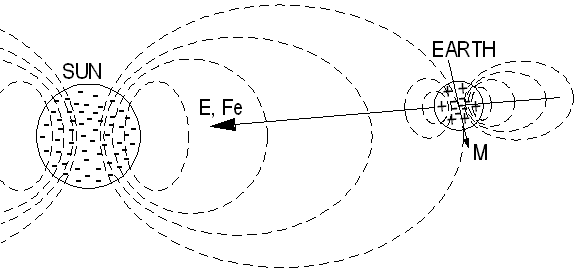Figure UA.5.1

Figure UA.5.1 shows the forces between the Sun and the Earth. The dashed line represents the magnetic field of the Sun. The arrow shows the electric field and the electrostatic force by the Sun. The ‘M’ is the magnetic moment of the Earth.

From the chapter ‘Sun’, we know that the Sun is a star. Stars are negatively charged. The strength of the M&E fields depends on the size of the stars. The Sun is a very high negative charged fire ball which produces a strong M&E field surrounding it.

Planets are dielectric and conductor. They can become electric dipole. The electric field by the Sun exerts a strong attractive force to the planets and causes the planets to circulate around it, including the Earth. The strong electric field by the Sun induces the charge separation on the Earth. In order to reduce the strength of the electric field of the Sun, the Earth is induced to positive charge on the surface and the negative charges are pushed into the core of the Earth as shown in figure UA.5.1. The higher the negative charge of the Sun, the stronger the M&E field of the Sun and the higher the positive charge induced on the surface of the Earth.

The attraction force between the Earth and the objects on the Earth is based on the same principle, where the positive charges on the surface of the Earth exerts an electrostatic force to all the objects on the Earth. All the objects on the Earth are appeared neutral relatively. The satellite of the Earth or the Moon is attracted by the Earth due to the electrostatic force from the Earth. More description about the charges distribution of the Earth is discussed in the chapter ‘Earth’.

Beside electrostatic force; the magnetic field of the Sun also exerts a magnetic force to the Earth. The magnetic force of the Sun causes a different effect on the Earth. In spite of pulling, the magnetic force also tilts the rotation axis of the Earth. The Earth is forced to tilt to the rotation angle where the magnetic moment of the Earth is aligned to the magnetic field of the Sun. This is the reason that the equator of the Earth faces the Sun, but not the North Pole or the South Pole.

UA.6.0        EFFECT OF KONG GRAVITATIONAL FORCE

For a rotating charged body like star, due to the difference in velocity and density of charges circulating in the star, the generated equal-potential magnetic field tends to flatten horizontally. The phenomenon is illustrated in figure UA.6.1 below.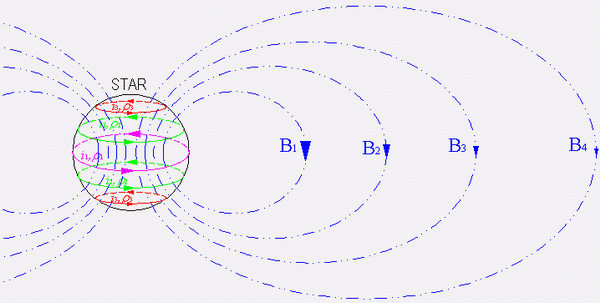Figure UA.6.1

From figure UA.6.1, the charges distribution in the star is as follow,

i1 > i2 > i3 and ρ1 > ρ2 > ρ3,                                                      … eq. UA.6.1

The current flow in the star is stronger at the equator of the star and gradually reduces to top and bottom. The density distribution on the charges is higher at the equator and gradually reduces to top and bottom of the star. Therefore, the magnetic field generated is stronger at the center and gradually reduce to top and bottom. The blue dashed line of figure UA.6.1 indicates the superposition of the equal-potential magnetic field generated. The superposition of the generated magnetic field is elongated and stretched outwards horizontally to form an ellipsoidal toroid.

The strength of the equal-potential magnetic field decreases when the distance from the star increases in horizontal plane. In vertical plane, the strength of the equal-potential magnetic field is the highest at the equator and gradually decreases to the top and bottom, where

B1 > B2 > B3 > B4                                                                    … eq. UA.6.2

Figure UA.6.2 below illustrate the effect of Kong gravitational force to the circulating object with magnetic dipole M.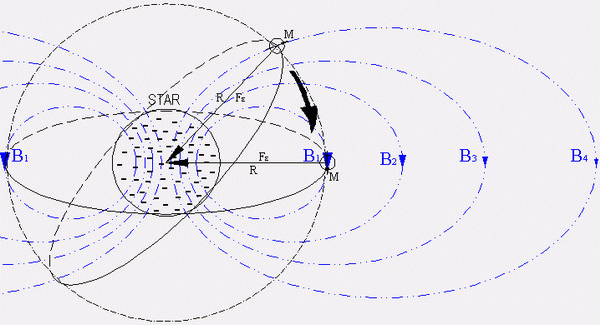Figure UA.6.2

When the object M travels near to the star, the electrostatic force FE will pull the object M. The pulling of centrifugal force causes the object to revolve at distance R around the star regardless of any direction as long as perpendicular to the star. If only base on the electrostatic force, the orbiting object has no preference to the tilting angle from the equator.

When the orbiting object is tilted to certain angle from the equation, the object will cut through different strength of the star’s magnetic field. The change in magnetic flux will create a force to topple the object; causing the object tends to orbit at the equator.

From eq. UA.2.20 below,

U = - m × Bext                                                                            … eq. UA.2.20

where   m = magnetic dipole

Bext = external magnetic field

If the magnetic dipole of the object is constant, the magnetic potential energy is depending on the strength of magnetic field. From eq. UA.6.2, the magnetic field of B1 possesses the highest strength. When Bext = B1, the system possesses the lowest magnetic potential energy. For any radius of circulation, the highest magnetic field is always at the equator. Hence, the object tends to circulate at the equator region of the star in order to achieve lowest energy.

The revolving velocity and the distance apart of the object are determined and dominated by the electrostatic force; while the angle or the position of the object is determined by the magnetic force.

Due to the interaction between the Kong gravitational force between stars and planets, the objects tend to circulate around the equator. The planets are attracted and revolving in planar, such as the Earth is circulating around the Sun as shown in figure UA.5.1.

Base on the same reason, where the Kong gravitational force governs the activities in the Universe, the solar system is planar in shape. Similarly, the circulations of matters in the Galaxy and the Universe are in planar. This also causes the Universe expands horizontally.

If the Universe is governed by the mass-dependent gravitational force, the Universe shall expand in spherical shape instead of planar, as the mass-dependent gravitational force has no preference in attracting matters from any directions, but electromagnetic force does. Where the Kong gravitational force is the actual force that governing all activities in the Universe.

UA.7.0        KONG GRAVITATIONAL FORCE IN GALAXIES

The Kong gravitational force plays very important role in determining the shape of the galaxies in this Universe. Many of the mature galaxies are planar or flat in shape where the Kong gravitational force keeps the orbiting objects at the equator of the galaxies. Figure UA.7.1 shows one of the galaxies that appeared in the Universe, which is the Andromeda galaxy. This galaxy is almost flat in shape. The center of rotation is the center attraction of the galaxy.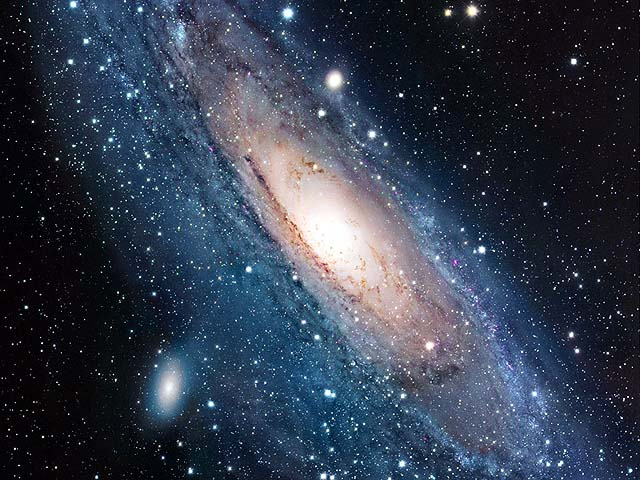Figure UA.7.1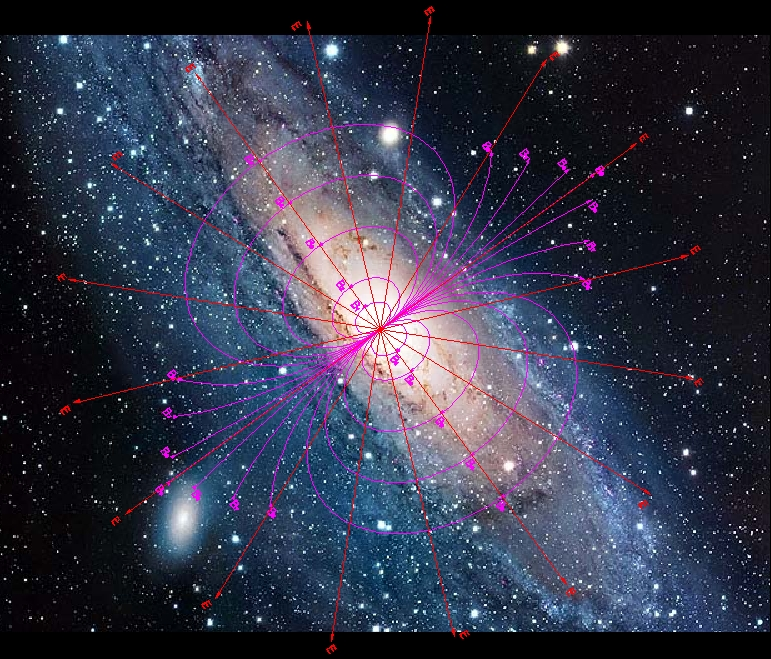Figure UA.7.2

Figure UA.7.2 illustrates the magnetic and electric field of the galaxy which governing the activity of the galaxy. The effect of electric force has longer distance while the effect of magnetic force has shorter distance. For an object that far from the center of attraction, electric force is dominant to magnetic force, the attracted object may not falls to the equator of the galaxy due to weak magnetic force.

UA.8.0        RELATIONSHIP BETWEEN KONG AND NEWTONIAN GRAVITATIONAL FORCE

Although electromagnetic force is playing the role of attraction force in the Universe, the electrostatic force is always dominant to magnetic force. The characteristic of electrostatic force is similar to mass-dependent gravitational force.

From eq. UA.4.7, we have,

FG = FE + FB                                                                          … eq. UA.4.7

where   FG = gravitational force

FE = electrostatic force

FB = magnetic force

In many cases, electrostatic force dominants magnetic force where the magnetic force is negligible. We can then relate the gravitational force and electrostatic force as follow,

FG = FE                                                                                   …eq. UA.7.1

Substituting eq. 3.1 and eq. 4.1 to the above, we obtain,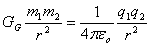… eq. UA.7.2

Mass is a function of charge and assuming the change in magnetic and time is negligible. The mass can be expressed as follow,

m = kq                                                                                    … eq. UA.7.3

By replacing the mass in eq. UA.7.2 with eq. UA.7.3, we obtain,… eq. UA.7.4

Rearrange eq. UA.7.4, the gravitational constant becomes,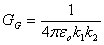… eq. UA.7.5

Both electrostatic and gravitational forces are interchangeable by adding constants. Although many theories had been developed by using the gravitational constant, these theories can be modified base on permittivity constant as shown in eq. UA.7.5.

DISCUSSIONS AND CONCLUSIONS

The mass dependent gravitational force is not the actual force that governs the activities in the Universe. The actual forces are the magnetic and electric forces, which is the Kong gravitational force. A mass is not able to produce the pulling force but a mass can be electrically charged and induced by external M&E field. A charged mass can produce the electrostatic and magnetic fields and forces which able to attract objects surrounding it.

Light is bent near a 'massive' object due to the Kong Vector of light that corresponds to the different pattern of the M&E field of the 'massive' object. It is not because the light is 'attracted' by the 'massive' object according to the mass dependent gravitational force. This will be discussed further in chapter "Kong matter and Kong Energy".

The uni-direction of Kong gravitational force is caused by the uni-direction of electrostatic force to a neutral object and the uni-direction of the magnetic force in order to achieve lower energy level.

In this Universe, it is observed that all the planets are circulating around the stars. The stars possess very high negative charge. The electrostatic force of the stars pulls the planets to circulate around them. This is the reason that the stars are always the center of rotation of all the planets. But it is hardly found that planets circulate surrounding planets.

The strength of the attraction force between the stars and the planets depends on the density of the charge of the stars. The higher the negative charge of the star, the star exerts the stronger attraction force to the planets. This magnitude of the M&E pulling force is different from one star to another star.

This M&E attraction force does not depend on the amount of mass, but the charging index (capacitance) of the planets determine the charge density to be induced by the stars. Due to the strong electric field surrounding the star, the planets are induced to produce the separation of the positive and negative charges, in order to weaken the strength of the electric field of the star.

For planets located far from the stars, the electrostatic force plays a major role in pulling the planets. For planets located near to the stars, both the electrostatic and magnetic forces are pulling the planets. The magnetic field of the Sun causes the tilting effect of the Earth and maintains the rotation axis of the Earth. This is the reason that the equator of the Earth faces the Sun, but not the North Pole or the South Pole.

More supportive explanations are presented in chapter ‘Earth’. It is described that the strong magnetic field of the Earth is produced by the rotation effect of the net positive and negative charges of the Earth. It is also described the reason that the lightning strikes on the Earth because the Earth is positive charged on the surface.

This website is originated on 15-Mar-2007,

updated on 4-Jan-2009.